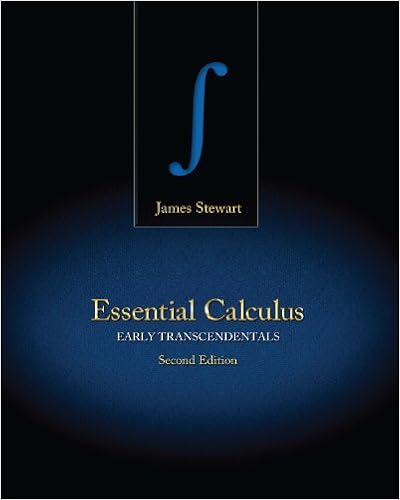# 260 Lab 7 - Math 260 Week 7 Lab Name Shelley Adkins...

• Test Prep
• ShelleyA7
• 16
• 67% (3) 2 out of 3 people found this document helpful

This preview shows page 1 - 3 out of 16 pages.

##### We have textbook solutions for you!
The document you are viewing contains questions related to this textbook.The document you are viewing contains questions related to this textbook.
Chapter 5 / Exercise 8
Essential Calculus: Early Transcendentals
StewartExpert Verified
Math 260 - Week 7 LabName:Shelley AdkinsCategory 1: Area Under a Curve Part IIRecall from Lab 6:0 < , if f(x) is integrable and above the x-axis on [a,b].That is, all areas are positive unless the area is beneath the x-axis. If the area is below the x-axis, it will be negative.If part of the area is above and part below, the integral will give the net area. To find the correct total area, it must be broken into separate integrals, one for the area above and one for below the x-axis.Also recall: = + , for a < c < bThat is, a region under a curve can be broken into two (or more) regions as long as the breaking point is within the region.1.) Find the area under the curve from [-3, 3] by using the Fundamental Rule of Calculus:. Show all work.
a.)Below is the graph of x3– 9x with the area between the graph and the x-axis from – 3 to 3 shaded. How does it help to explain your answer to a.) above ?
##### We have textbook solutions for you!
The document you are viewing contains questions related to this textbook.The document you are viewing contains questions related to this textbook.
Chapter 5 / Exercise 8
Essential Calculus: Early Transcendentals
StewartExpert Verified
b.)What must be done to determine the area using the Fundamental Theorem of Calculus ?= ?
c.)Find the value of the definite integrals 1 & 2 below, then use the results to find 3.
0
•••